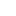Share:

# MathCommon Core State Standards

Math Common Core State Standards require greater focus by teachers and deeper knowledge by students than many previous state standards. Students will need to accurately calculate equations, understand concepts not just memorize answers, and accurately select the best mathematical concept or equation to solve real-world problems, while demonstrating why the method or equation they selected is accurate. The new standards make sure students are learning and absorbing the critical information they need to succeed at higher levels.

### Elementary School Example

Previous Math Question Common Core Math Question
Each shirt costs \$4. How much do 3 shirts cost? Each shirt has 6 buttons. How many buttons are needed to make 7 shirts?
This question can be answered by a “count-all” strategy, in which you don’t need to know your multiplication tables by memory to get the right answer. This question requires automatic recall of multiplication tables to get at the right answer.

### Middle School Example #1

Previous Math Question Common Core Math Question
Donna buys 40 apples at 35 cents each. She eats 2 apples and sells the rest for 45 cents each. How much money does she make? Donna buys some apples at 35 cents each. She eats 2 apples and sells the rest for 45 cents each. She makes \$4.40. How many apples did she buy?
This question only requires use of simple arithmetic. This question requires use of an algebraic equation.

### Middle School Example #2

Previous Math Question Common Core Math Question
A bird flew 20 miles in 100 minutes at constant speed. At that speed, how long would it take the bird to fly 6 miles? A bird flew 20 miles in 100 minutes at constant speed. At that speed: (a) how long would it take the bird to fly 6 miles? (b) How far would the bird fly in 15 minutes? (c) How fast is the bird flying in miles per hour? (d) What is the bird’s pace in minutes per mile?
This question requires one calculation, using a formula. This question requires a series of calculations and reasoning. It measures if students understand why the formula works.

### High School Example

Previous Math Question Common Core Math Question
If 3(y-1) = 8, then what is y? What are two different equations with the same solution as 3(y-1) = 8?
This question is an example of solving equations as a series of mechanical steps. This question is an example of solving equations as a process of reasoning.

Article Source: ExcelInEd

### Bartlett Online Tutoring

Club Z! of Bartlett offers Online Tutoring and Test Prep for most subject areas and grade levels!

### Contact Club Z! of Bartlett